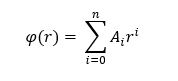# Point Imaging with a Radial Polynomial Grating

The Radial Polynomial Phase Departure option on a Two-point holographic optical element grating definition allows for radial phase (OPL) to be added to a grating definition using the following form:Taking the convention for a standard y-z "layout" view, that z-positions left of a surface are negative and z positions to the right a surface are positive, consider and object and image point located at d and d' relative to the grating surface.  The refractive index values of the object and image points are n and n', respectively.

The Radial Phase polynomial can be used to implement the parabolic approximation to point imaging in this configuration by setting the A_2 coefficient to a value of 0.5*(n'/d' - n/d) and using a diffraction order of -1.

For example, consider an object point located at z = -25 mm and an image point located at z = +50 mm (both points immersed in air). The A_2 coefficient value would be 0.5*(1/50 - (-1/25)) = 0.03.  This configuration is shown in the example file below.